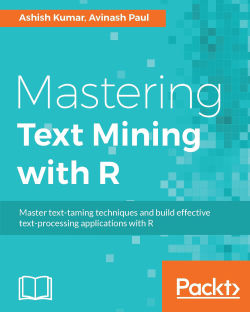# Mastering Text Mining with R4.5 (2 reviews total)
By Ashish Kumar , Avinash Paul
• FREE Subscription Access now
• \$10.00 Was 31.99 eBook
What do you get with a Packt Subscription?

• Constantly updated with 100+ new titles each month
• Breadth and depth in over 1,000+ technologies

Text Mining (or text data mining or text analytics) is the process of extracting useful and high-quality information from text by devising patterns and trends. R provides an extensive ecosystem to mine text through its many frameworks and packages.

Starting with basic information about the statistics concepts used in text mining, this book will teach you how to access, cleanse, and process text using the R language and will equip you with the tools and the associated knowledge about different tagging, chunking, and entailment approaches and their usage in natural language processing. Moving on, this book will teach you different dimensionality reduction techniques and their implementation in R. Next, we will cover pattern recognition in text data utilizing classification mechanisms, perform entity recognition, and develop an ontology learning framework.

By the end of the book, you will develop a practical application from the concepts learned, and will understand how text mining can be leveraged to analyze the massively available data on social media.

Publication date:
December 2016
Publisher
Packt
Pages
258
ISBN
9781783551811

## Chapter 1. Statistical Linguistics with R

Statistics plays an important role in the fields that deal with quantitative data. Computational linguistics is no exception. The quantitative investigation of linguistic data helps us understand the latent patterns that have helped phoneticians, psycholinguistics, linguistics, and many others to explore and understand language.

In this chapter, we will explain the basic terms associated with probability, used in computational linguistics. You will soon get to dive into linguistics and learn about language models and practical quantitative methods used in linguistics.

At the end of this chapter, we will extensively discuss some very useful and highly efficient packages in R, which we will use throughout this book, and by the time you finish the book, you should be able to pick appropriate R packages and functions for specific text-mining activities and be able to effectively use them for practical purposes.

In this chapter, we will cover the following topics:

• Basic statistics and probability

• Probabilistic linguistics

• Language models

• Quantitative methods in linguistics

• R packages for text mining

## Probability theory and basic statistics

The conceptual origin of statistics is perceived to be from probability theories. We all must have heard something like the probability of rain tomorrow is 50%. While this seems very quantitative and thus should be easily interpretable, it is not very clear what it means. It can be interpreted to mean that for all the days when weather conditions are the same as tomorrow, it will rain on half of those days.

Probability helps us calculate the extent to which something is likely to happen or the likelihood of an event.

Probability is useful in various fields, such as statistics, computer science, physics, finance, gambling, sports, medicine, and even in machine learning and artificial intelligence.

### Probability space and event

Probability in mathematics is built around sets. Set theory is very useful in probability; it provides a language for expressing and working with events.

The sample space of an experiment is the set of all possible outcomes of the experiment; let's call it S. An event, let's call it A, is a subset of the sample space S, and we say that A occurred if the actual outcome is in A.

Let's take an example of picking a card from a standard deck of 52 cards. The sample space S is the set of all the cards. Let's us consider an event A where the card we pick is an ace. This is a subset of the sample space. So the probability P of picking an ace is:

Probability = (number of elements in the event) / (number of elements in the sample space).

### Theorem of compound probabilities

This says that the probability of the intersection of two events A and B can be computed as the product of probability of A given that B has happened times the probability of B: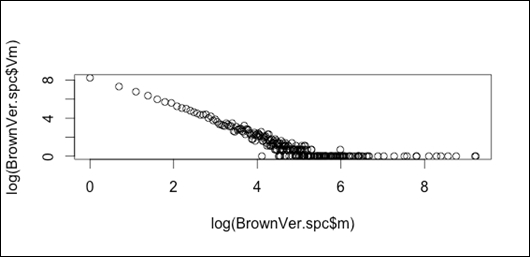The law of total probability or law of alternatives can be formulated as follows: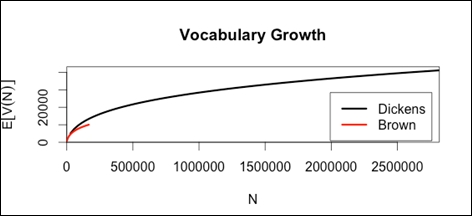### Conditional probability

Probability is a way of expressing uncertainties about events. Whenever we observe new evidence or obtain data, we acquire information that may affect our uncertainties. Conditional probability is the concept that tells us how to express the probability which is affected by the newly acquired information. Conditional probability handles situations where you have some additional knowledge about the outcome of a trial or experiment.

Let's consider an event R, It will rain today, before looking at the sky. The probability P(R) will increase when we look at the sky and see dark clouds. So the new probability is P (R|C) where C is the event of dark clouds.

If A and B are events with P (B) > 0,then the conditional probability of A given B, denoted by P(A|B), is defined as: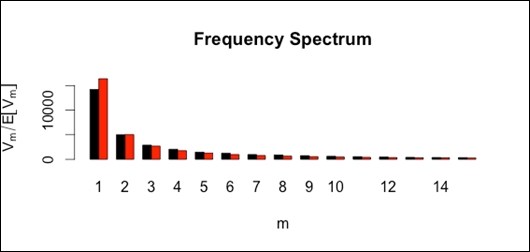Let us consider an example and try to perform the same using R. We are rolling two dice and the objective is to find the probability of the sum of the outcomes being greater than or equal to 8, given that the first dice has resulted in 3:

```library(prob)
S <- rolldie(2, makespace = TRUE)
A <- subset(S, X1 + X2 >= 8)
B <- subset(S, X1 == 3) #Given
Prob(A, given = B) ```

### Bayes' formula for conditional probability

Bayes' formula gives us a way to test a hypothesis using conditional probabilities. A hypothesis is a suggested explanation for a specific outcome. If we see that a probability P (A | B) is high, we might hypothesize that event B is a cause of the event A. We use Bayes' formula when we know conditional probabilities of the form P (B | A) and want a conditional probability of the form P (A | B):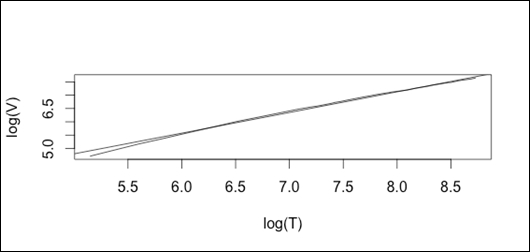### Independent events

Two events, A and B, in the same sample space are independent if P (AB) = P (A) P (B).This formula gives us a new and simpler way to characterize independent events. Two events, A and B, are independent if the probability of both events happening together is equal to the product of the probabilities of the two events.

### Random variables

In probability, a random variable is a rule or function that assigns a number to each element of a sample space. In other words, a random variable gives a number for each outcome of a random experiment. In statistics, we define random variables using the letter X. There are different types of random variable.

### Discrete random variables

When we toss two coins, the number of heads we can get is 0, 1, or 2 .We can define X as the number of heads that I get during this experiment. These random variable values have a probability associated with them; these variables can be represented as discrete points on a number line so they are called discrete random variables.

#### Continuous random variables

Let's say that we have to look at the physics test scores of 100 class 10 students. The test scores will fall between 0% and 100%. The test scores of the students may vary, such as 95.5%, 88%, 97.2%, and so on. We cannot denote all the test scores using discrete numbers when all values in an interval are possible. This is called a continuous random variable.

### Probability frequency function

Once we have a random variable, we can determine the probability that the random variable will have a certain value; for example, for rolling two dice to get a sum of five outcomes, it can be (1,4) , (4,1) , (3,2) , or (2,3) so there are 4 out of 36 possible outcomes, so: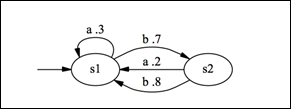### Probability distributions using R

R provides a wide range of probability functions. The generic prefixes for probability functions in R are r, d, p, q, for random number generators, probability density function, cumulative density function, and quantile function, respectively.

A comprehensive list of functions available is as follows:

Distribution

Functions

Beta

`pbeta`

`qbeta`

`dbeta`

`rbeta`

Binomial

`pbinom`

`qbinom`

`dbinom`

`rbinom`

Cauchy

`pcauchy`

`qcauchy`

`dcauchy`

`rcauchy`

Chi-Square

`pchisq`

`qchisq`

`dchisq`

`rchisq`

Exponential

`pexp`

`qexp`

`dexp`

`rexp`

F

`pf`

`qf`

`df`

`rf`

Gamma

`pgamma`

`qgamma`

`dgamma`

`rgamma`

Geometric

`pgeom`

`qgeom`

`dgeom`

`rgeom`

Hypergeometric

`phyper`

`qhyper`

`dhyper`

`rhyper`

Logistic

`plogis`

`qlogis`

`dlogis`

`rlogis`

Log Normal

`plnorm`

`qlnorm`

`dlnorm`

`rlnorm`

Negative Binomial

`pnbinom`

`qnbinom`

`dnbinom`

`rnbinom`

Normal

`pnorm`

`qnorm`

`dnorm`

`rnorm`

Poisson

`ppois`

`qpois`

`dpois`

`rpois`

Student t

`pt`

`qt`

`dt`

`rt`

Studentized Range

`ptukey`

`qtukey`

`dtukey`

`rtukey`

Uniform

`punif`

`qunif`

`dunif`

`runif`

Weibull

`pweibull`

`qweibull`

`dweibull`

`rweibull`

Wilcoxon Rank Sum Statistic

`pwilcox`

`qwilcox`

`dwilcox`

`rwilcox`

Wilcoxon Signed Rank Statistic

`psignrank`

`qsignrank`

`dsignrank`

`rsignrank`

### Cumulative distribution function

This frequency function gives the probabilities for each value in the range of a random variable. For a given value R of the random variable, the cumulative distribution function gives the probability of the random variable taking on a value up to and including the given value R. When R is 3, there are three outcomes, (1, 1), (1, 2), and (2, 1), so: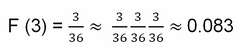The cumulative distribution function is also called the CDF, or probability distribution or distribution function. The stats package in R provides the function `ecdf` to compute the empirical cumulative distribution function and plot it using the object created. You can also plot the `ecdf` object using the `ggplot2` package. Let's look at an example for the same:

```x <- rnorm(1000, 99.2, 1.2)
y <- rnorm(10000, 97.3, 0.85)
z <- rnorm(10000, 98.1, 0.4)

# Create a chart with all 3 Conditional distribution plots
plot(ecdf(x), col=rgb(1,0,0), main=NA)

# Adding legend to the chart.
legend('right', c('x', 'y', 'z'), fill=c(rgb(1,0,0), rgb(0,1,0), rgb(0,0,1)))
```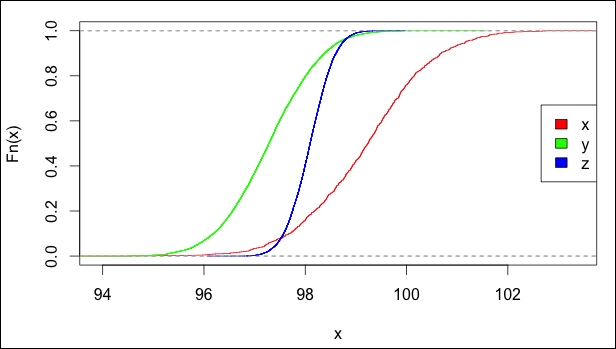Using the `ggplot2` package, create the CDF plot with the `ecdf` function:

```  # Load the required packages.
library("reshape2","plyr","ggplot2")

# transform the data.
plot_data <- melt(data.frame(x, y, z))
plot_data <- ddply(plot_data, .(variable), transform, cd=ecdf(value)(value))

# Create the CDF using ggplot.
cdf <- ggplot(plot_data, aes(x=value)) + stat_ecdf(aes(colour=variable))

# Generate the Conditional distribution plot.
cdf
```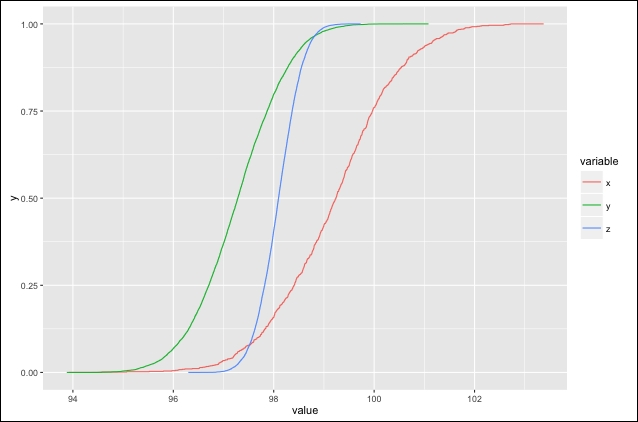### Joint distribution

Two different random variables can be associated with the same sample space. When there are two random variables on the same sample space, we study their interaction using a joint distribution. Let's consider an example: we want to know the probability that the sum of the same dice rolled twice is 6, so S = 6, and that the lowest die is 3, so D = 3. We represent this as follows:

P{S = 6, D = 3}

P {S = 6} = (1, 5) (2, 4) (3, 3) (4, 2) (1, 5);

Of the five outcomes, only one has the lower number equal to 3 so the probability is: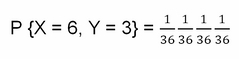### Binomial distribution

If there are only two outcomes to a trial, one with probability P and the other with probability 1 – P, often one outcome is called a success and the other a failure. When this is the case, P is used as the probability of success and the probability of failure is 1 – P. Such an experiment is called a Bernoulli trial or a binomial trial, because there are only two outcomes. The random variable associated with a Bernoulli trial is the Bernoulli random variable, with value 1 for a successful outcome and value 0 for failure.

Let's take an example of flipping a coin. It gives two outcomes, heads and tails. If we assign the value 1 to heads and 0 to tails, we have a Bernoulli random variable. Let's call this random variable R and since heads and tails are equally likely to occur:

P{R = 1} = 0.5 and P{R = 0} = 0.5

If we repeat a Bernoulli trial many times over, we get a new distribution, called a binomial distribution. So in order to compute the probability of k successes in n trials we can use the following formula: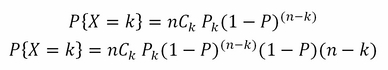Here:

• n: Number of trials

• P: Probability of success

### Poisson distribution

The Poisson distribution applies when occurrences are independent, so that one occurrence neither diminishes nor increases the chance of another. The average frequency of occurrence for the time period is known. The probability of an occurrence during a small time interval is proportional to the entire length of the time interval: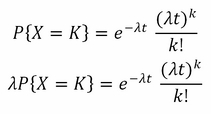Here:

• λ: Average rate of outcomes

•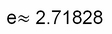• t: Interval size

### Counting occurrences

When we are putting together texts, we will not know the probability distribution of a particular topic. If we consider a corpus of country's economic strategy, written by various economists, it's difficult to understand the probability of what they are emphasizing more – is it infrastructure, manufacturing, banking, and so on – without counting the members associated. One thing to be aware of is no corpus will be balanced. We need to count the occurrences of relevant words in the dataset to get some statistical information. We need to know the frequency distribution of different words. Word frequencies refer to the number of word tokens that are instances of a word type. We can perform word counts over corpora with the R tau package.

### Zipf's law

Zipf's law is an interesting phenomenon that can be applied universally in many contexts, such as social sciences, cognitive sciences, and linguistics. When we consider a variety of datasets, there will be an uneven distribution of words. Zipf's law says that the frequency of a word, f (w), appears as a nonlinearly decreasing function of the rank of the word, r (w), in a corpus. This law is a power law: the frequency is a function of the negative power of rank. C is a constant that is determined by the particulars of the corpus; it's the frequency of the most frequent word: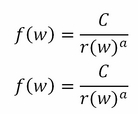Given a collection of words, we can estimate the frequency of each unique word, which is nothing but the number of times the word occurs in the collection.

If we sort the words in descending order of their frequency of occurrence in the collection, and compute their rank, the product of their frequency and associated rank reveals a very interesting pattern.

• N: Sample size or corpus size

• V: Vocabulary size, count of distinct type in the corpus

• Vm: Count of hapax terms, types that occur just once in a corpus

Let us consider a small sample S: `a a a a b b b c c d d`:

1. Here, N= 11, V = 4, Vm = 0.

2. Load Brown and Dickens frequency data:

```library(zipfR)
data(Dickens.spc)
data(BrownVer.spc)```
3. Check sample size and vocabulary and hapax counts:

```N(BrownVer.spc)          # 166262
V(BrownVer.spc)          # 10007
Vm(BrownVer.spc,1)       # 3787
N(Dickens.spc)           # 2817208
V(Dickens.spc)           # 41116
Vm(Dickens.spc,1)        # 14220```
4. Zipf rank-frequency plot:

`plot(log(BrownVer.spc\$m),log(BrownVer.spc\$Vm))`5. Compute binomially interpolated growth curves:

```di.vgc <- vgc.interp(Dickens.spc,(1:100)*28170)
br.vgc <- vgc.interp(BrownVer.spc,(1:100)*1662)```
6. Plot vocabulary growth:

`plot(di.vgc,br.vgc,legend=c("Dickens","Brown"))`7. Compute Zipf-Mandelbrot model from Dickens data:

```zm <- lnre("zm",Dickens.spc)
## plot observed and expected spectrum
zm.spc <- lnre.spc(zm,N(Dickens.spc))```Let there be a word w which has the rank r' in a document and the probability of this word to be at rank r' be defined as P(r'). The probability P(r') can be expressed as the function of frequency of occurrence of the words as follows:

`P(r') = Freq(r')/N,`

where N is the sample size and Freq(r') is the frequency of occurrence of r' in the corpus

### Note

As per Zipf's law, r' * P(r') = K, where K is a constant. The value of K is assumed to be close to 0.1.

### Heaps' law

Heaps' law is also known as Herdan's Law. This law was discovered by Gustav Herdan, but the law is sometimes attributed to Harold Heaps. It is an empirical law which describes the relationship between type and tokens in linguistics. In simpler terms, Heaps' law defines the relation between the count of distinct words in document and the length of the specified document.

The relation can be expressed as:

Vr(n) = C* nb

Here, Vr is the count of distinct words in document and n is the size of the document. C and b are parameters defined empirically.

The similarity between Heaps' Law and Zipf's law is attributed to the fact that type-token relation is derivable from type distribution:

```library(tm)
data("acq")
termdoc <- DocumentTermMatrix(acq)
Heaps_plot(termdoc)
```### Lexical richness

Quantitative analysis of lexical structure is relevant for many activities such as stylometrics, applied linguistics, computational linguistics, natural language processing, lexicology, and so on. There are different approaches to capture vocabulary richness. It can be measured by means of measure or of an index. It can be captured by means of curve, as in the case of Herdan's and Zipf's law. If we consider the empirical distribution of word types, we can derive the distribution based on combinatorial considerations or we can use consider the stochastic processes to derive the distribution.

In applied linguistics, lexical richness explains the qualified proficiency of the author in a document, in terms of language variation, width, length, and productive knowledge of vocabulary. Let's attempt to understand the multiple measures that explain the lexical richness of a text.

### Note

The languageR package in R comes with functions to compare lexical richness between corpora.

#### Lexical variation

Lexical variation in language is considered to be multi-dimensional; all languages go through variations based on time and social settings. There are different lexical variants to the same word in same language. For instance, in the US, what you call a cookie is a biscuit in the UK. Most of us are aware of language variation based on geographical differences, such as elevator and lift, pavement and sidewalk, pants and trousers. Socio-cultural changes lead to the phenomenon of borrowing in cases of dialect contacts. Semantic shifts and broadening give the words different meanings in different contexts. While by semantic broadening, the words take a more generalized meaning, by semantic narrowing, it is bound to take more restricted meaning. Broadly, lexical variations are of two categories: conceptual variation and contextual variation, which is further categorized to formal variation, semasiologically variation, and onomasiolofical variation.

### Note

The koRpus package in R provides functions to estimate lexical variation.

#### Lexical density

Lexical density is defined as the ratio of content to functional or grammatical words in a sentence. It is used in discourse analysis for texts. In simpler terms, lexical density explains the readability of a text.

Lexical density is determined as follows:

`              Ld  = (Nlex / N) * 100`

Here:

• Ld = Lexical density

• Nlex = Count of lexical tokens

• N = Count of all tokens

#### Lexical originality

Lexical originality measures the unique wording of a specific writer. It is defined as the number of unique word types*100/total lexical words.

#### Lexical sophistication

Lexical sophistication measures the percentage of advanced words in text. It is defined as the (number of advanced lexemes)*100 /(total number of lexical words).

For identifying single word lexemes, we can use the technique of stemming.

## Language models

In terms of natural language processing, language models generate output strings that help to assess the likelihood of a bunch of strings to be a sentence in a specific language. If we discard the sequence of words in all sentences of a text corpus and basically treat it like a bag of words, then the efficiency of different language models can be estimated by how accurately a model restored the order of strings in sentences. Which sentence is more likely: I am learning text mining or I text mining learning am? Which word is more likely to follow I am…?

Language models are widely used in machine translation, spelling correction, speech recognition, text summarization, questionnaires, and so on.

Basically, a language model assigns the probability of a sentence being in a correct order. The probability is assigned over the sequence of terms by using conditional probability. Let us define a simple language modeling problem. Assume a bag of words contains words W1, W2,………………….,Wn.. A language model can be defined to compute any of the following:

• Estimate the probability of a sentence S1: P (S1) = P (W1, W2, W3, W4, W5)

• Estimate the probability of the next word in a sentence or set of strings:

`P (W3|W2, W1)`

How to compute the probability? We will use chain law, by decomposing the sentence probability as a product of smaller string probabilities:

`            P(W1W2W3W4) = P(W1)P(W2|W1)P(W3|W1W2)P(W4|W1W2W3)`

### N-gram models

N-grams are important for a wide range of applications. They can be used to build simple language models. Let's consider a text T with W tokens. Let SW be a sliding window. If the sliding window consists of one cell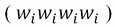then the collection of strings is called a unigram; if the sliding window consists of two cells, the output is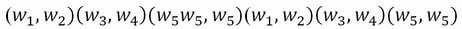, this is called a bigram .Using conditional probability, we can define the probability of a word having seen the previous word; this is known as bigram probability. So the conditional probability of an element, , given the previous element,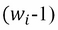is: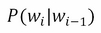Extending the sliding window, we can generalize that n-gram probability as the conditional probability of an element given previous n-1 element: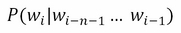The most common bigrams in any corpus are not very interesting, such as on the, can be, in it, it is. In order to get more meaningful bigrams, we can run the corpus through a part-of-speech (POS) tagger. This would filter the bigrams to more content-related pairs such as infrastructure development, agricultural subsidies, banking rates; this can be one way of filtering less meaningful bigrams.

A better way to approach this problem is to take into account collocations; a collocation is the string created when two or more words co-occur in a language more frequently. One way to do this over a corpus is pointwise mutual information (PMI).The concept behind PMI is for two words, A and B, we would like to know how much one word tells us about the other. For example, given an occurrence of A, a, and an occurrence of B, b, how much does their joint probability differ from the expected value of assuming that they are independent. This can be expressed as follows: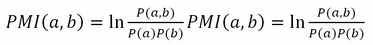• Unigram model:

`              Punigram(W1W2W3W4) = P(W1)P(W2)P(W3)P(W4)`
• Bigram model:

```              Pbu(W1W2W3W4) = P(W1)P(W2|W1)P(W3|W2)P(W4|W3)
P(w1w2…wn ) = P(wi | w1w2…wi"1)```

Applying the chain rule on n contexts can be difficult to estimate; Markov assumption is applied to handle such situations.

### Markov assumption

If predicting that a current string is independent of some word string in the past, we can drop that string to simplify the probability. Say the history consists of three words, Wi, Wi-1, Wi-2, instead of estimating the probability P(Wi+1) using P(Wi,i-1,i-2) , we can directly apply P(Wi+1 | Wi, Wi-1).

### Hidden Markov models

Markov chains are used to study systems that are subject to random influences. Markov chains model systems that move from one state to another in steps governed by probabilities. The same set of outcomes in a sequence of trials is called states. Knowing the probabilities of states is called state distribution. The state distribution in which the system starts is the initial state distribution. The probability of going from one state to another is called transition probability. A Markov chain consists of a collection of states along with transition probabilities. The study of Markov chains is useful to understand the long-term behavior of a system. Each arc associates to certain probability value and all arcs coming out of each node must have exhibit a probability distribution. In simple terms, there is a probability associated to every transition in states:

.Hidden Markov models are non-deterministic Markov chains. They are an extension of Markov models in which output symbol is not the same as state. We will discuss this topic in detail in later chapters.

## Quantitative methods in linguistics

Text can be grammatically complex, to analyze text its difficult consider all the complexity. In order to get meaning out of text or a document, we need a measure. We need to extract quantitative data by processing the text with various transformation methods. Each method discards unnecessary, ancillary information. There are various methods, packages, APIs, and software that can transform text into quantitative data, but before using any of them, we need to analyze and test our documents with different approaches.

The first step is we assume a document is a collection of words where order doesn't influence our analysis. We consider unigrams; for some analysis, bigrams and trigrams can also be used to provide more meaningful results. Next, we simplify the vocabulary by passing the document through a stemming process; here, we reduce the words to their root. A better/advanced approach would be lemmatization. Then we discard punctuation, capitalization, stop words, and very common words. Now we use this text for quantitative analysis. Let me list a few quantity methods and explain why they are used.

### Document term matrix

In order to find the similarity between documents in a corpus, we can use a document term matrix. In a document term matrix, rows represent documents, columns represent terms, and each cell value is the term frequency count for a document. It is one of the useful ways of modeling documents. Here is an example:

• Document-1: Ice creams in summer are awesome

• Document-2: I love ice creams in summer

• Document-3: Ice creams are awesome all season

icecream

summer

love

awesome

season

Doc1

1

1

0

1

0

Doc2

1

1

1

0

0

Doc3

1

0

0

1

1

If we visualize this in a term-document space, each document becomes a point in it. We can then tell how similar two documents are by calculating the distance between the two points using Euclidean distance.

When a term occurs in a lot of documents, it tends to make notably less difference the terms that occur few times. For example, India Today has more to do with India than today. These frequently occurring terms can affect the similarity comparison. The term space will be biased towards these terms. In order to address this problem, we use inverse document frequency.

#### Inverse document frequency

A commonly used measure of a term's selective potential is calculated by its inverse document frequency (IDF). The formula for IDF is calculated as follows: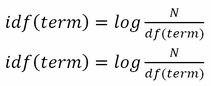Here, N is the number of documents in the `corpus` and `df(term)` is the number of documents in which the term appears.

The weight of a term's appearance in a document is calculated by combining the term frequency (TF) in the document with its IDF: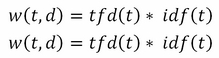This term–document score is known as TF*IDF, and is widely used. This is used by a lot of search platforms/APIs, such as SOLR, Elasticsearch, and lucene. TF*IDF scores are then pre-computed and stored, so that similarity comparison can be done by just a dot product.

When we look at the entries in this term–document matrix, most of the cells will be empty because only a few terms appear in each document; storing all the empty cells requires a lot of memory and it contributes no value to the dot product (similarity computation). Various sparse matrix representations are possible and these are used to for optimized query processing.

#### Words similarity and edit-distance functions

In order to find a similarity between words in case of fuzzy searches, we need to quantify the similarity between words; some quantitative methods used are explained below. Before going into it, let's install an R package, `stringdist`, which can be used to apply various algorithms mentioned above to calculate string similarity:

```install.packages("stringdist")
library(stringdist)
```

One way of finding the similarity between two words is by edit distance. Edit distance refers to the number of operations required to transform one string into another.

#### Euclidean distance

Euclidean distance is the distance between two points in the term-document space; it can be calculated by using the formula for a two-dimensional space as follows:

`Euclidean distance e <- sqrt((x1-x2)^2+(y1-y2)^2)`

Here, (x1,y1) and (x2,y2) are the two points and e is the estimated Euclidean distance between them: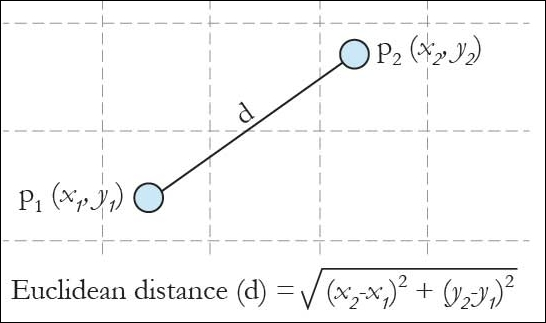We can very easily convert the aforesaid formula into R code:

```euclidean.dist <- function(x1, x2) sqrt(sum((x1 - x2) ^ 2))
```

#### Cosine similarity

Euclidean distance has its own pitfalls, documents with lots of terms are far from origin, we will find small documents relatively similar even if it's unrelated because of short distance.

To avoid length issues, we can use the angular distance and measure the similarity by the angle between the vectors; we measure the cosine of the angle. The larger the cosine value, the more similar the documents are. Since we use the cos function, this is also called cosine similarity: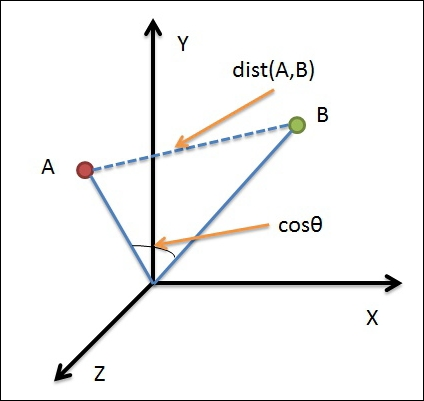The formula to calculate cosine between two points is as follows: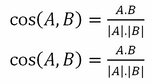This kind of geometric modeling is also called vector space model:

```# Create two random matrices matrixA and matrixB
ncol<-5
nrow<-5
matrixA<-matrix(runif(ncol*nrow), ncol=ncol)
matrixB<-matrix(runif(ncol*nrow), ncol=ncol)

# function for estimating cosine similarity in R:
cosine_sim<-function(matrixA, matrixB){
m=tcrossprod(matrixA, matrixB)
c1=sqrt(apply(matrixA, 1, crossprod))
c2=sqrt(apply(matrixB, 1, crossprod))
m / outer(c1,c2)
}

# Estimate the cosine similarity between the two matrices initiated earlier
cosine_sim(matrixA,matrixB)
```

Alternately, cosine similarity can also be estimated by functions available in the packages lsa, proxy, and so on.

#### Levenshtein distance

The Levenshtein distance between two words, x and y, is the minimal number of insertions, deletions, and replacements needed for transforming word x into word y.

If we to convert abcd to abdc, we need to replace c with d and replace d with c so the distance is 2:

```Library(stringdist)
stringdist('abcd', 'abdc', method='lv')
 2
```

#### Damerau-Levenshtein distance

The Damerau-Levenshtein distance is the minimal number of insertions, deletions, replacements, and adjacent transpositions needed for transforming word x into word y.

If we to convert abcd to abdc, we need to swap c and d so the distance is 1:

```stringdist('abcd', 'abdc', method='dl')
 1```

#### Hamming distance

The Hamming distance between two words is the number of positions at which the characters are different. It is the minimum number of substitutions required to change into word into another. In order to use the Hamming distance, the words must be of the same length.

If we to convert abcd to abdc, we need to substitute c with d and d with c so the distance is 2:

```stringdist('abcd', 'abdc', method='hamming')
 2```
##### Jaro-Winkler distance

The Jaro-Winkler distance measure is best suited for short strings such as name comparison or record linkage. It is designed to compare surnames and names. The higher the distance, the more similar the strings in comparison are.

In order to measure the Jaro distance, we need to perform the following two tasks:

• Compute the number of matches

• Compute the number of transpositions

The Winkler adjustment involves a final rescoring based on an exact match score for the initial characters of both words. It uses a constant scaling factor P:

```stringdist('abcd', 'abdc' , method = 'jw' , p=0.1)
 0.06666667```
##### Measuring readability of a text

Readability is the ease with which a text can be read by a reader. The readability of a text depends on its content and the complexity of its vocabulary and syntax. There are a number of methods to measure the readability of a text. Most of them are based on correlation analysis, where researchers have selected a number of text properties (such as words per sentence, average number of syllables per word, and so on) and then asked test subjects to grade the readability of various texts on a scale. By looking at the text properties of these texts, it is possible to correlate how much "words per sentence" influences readability.

### Note

The `koRpus` package in R provides a hyphen function to estimate the readability of a given text.

#### Gunning frog index

The Gunning fog index is one of the best-known methods that measure the level of reading difficulty of any document. The fog index level translates the number of years of education a reader needs in order to understand the given material. The ideal score is 7 or 8; anything above 12 is too hard for most people to read.

The Gunning fog index is calculated as shown in the following steps:

1. Select all the sentences in a passage of approximately 100 words.

2. We need to calculate the average sentence length by doing a simple math of dividing number of words by number of sentences.

3. Count all the words with three or more syllables. Generally, words with more than three syllables are considered to be complex.

4. Sum up the average sentence length and the percentage of complex words.

5. Multiply the result by 0.4.

The formula is as shown here: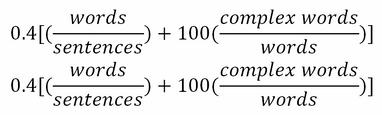## R packages for text mining

There is a wide range of packages available in R for natural language processing. Some of them are as follows.

### OpenNLP

OpenNLP is an R package which provides an interface, Apache OpenNLP, which is a machine-learning-based toolkit written in Java for natural language processing activities. Apache OpenNLP is widely used for most common tasks in NLP, such as tokenization, POS tagging, named entity recognition (NER), chunking, parsing, and so on. It provides wrappers for Maxent entropy models using the Maxent Java package.

It provides functions for sentence annotation, word annotation, POS tag annotation, and annotation parsing using the Apache OpenNLP chunking parser. The Maxent Chunk annotator function computes the chunk annotation using the Maxent chunker provided by OpenNLP.

The Maxent entity annotator function in R package utilizes the Apache OpenNLP Maxent name finder for entity annotation. Model files can be downloaded from http://opennlp.sourceforge.net/models-1.5/. These language models can be effectively used in R packages by installing the OpenNLPmodels.language package from the repository at http://datacube.wu.ac.at.

### Rweka

The RWeka package in R provides an interface to Weka. Weka is an open source software developed by a machine learning group at the University of Wakaito, which provides a wide range of machine learning algorithms which can either be directly applied to a dataset or it can be called from a Java code. Different data-mining activities, such as data processing, supervised and unsupervised learning, association mining, and so on, can be performed using the RWeka package. For natural language processing, RWeka provides tokenization and stemming functions. RWeka packages provide an interface to Alphabetic, NGramTokenizers, and wordTokenizer functions, which can efficiently perform tokenization for contiguous alphabetic sequence, string-split to n-grams, or simple word tokenization, respectively.

### RcmdrPlugin.temis

The `RcmdrPlugin.temis` package in R provides a graphical integrated text-mining solution. This package can be leveraged for many text-mining tasks, such as importing and cleaning a corpus, terms and documents count, term co-occurrences, correspondence analysis, and so on. Corpora can be imported from different sources and `analysed` using the `importCorpusDlg` function. The package provides flexible data source options to import corpora from different sources, such as text files, spreadsheet files, XML, HTML files, Alceste format and Twitter search. The Import function in this package processes the corpus and generates a term-document matrix. The package provides different functions to summarize and visualize the corpus statistics. Correspondence analysis and hierarchical clustering can be performed on the corpus. The `corpusDissimilarity` function helps analyse and create a cross-dissimilarity table between term-documents present in the corpus.

This package provides many functions to help the users explore the corpus. For example, `frequentTerms` to list the most frequent terms of a corpus, `specificTerms` to list terms most associated with each document, `subsetCorpusByTermsDlg` to create a subset of the corpus. Term frequency, term co-occurrence, term dictionary, temporal evolution of occurrences or term time series, term metadata variables, and corpus temporal evolution are among the other very useful functions available in this package for text mining.

### tm

The tm package is a text-mining framework which provides some powerful functions which will aid in text-processing steps. It has methods for importing data, handling corpus, metadata management, creation of term document matrices, and preprocessing methods. For managing documents using the tm package, we create a corpus which is a collection of text documents. There are two types of implementation, volatile corpus (VCorpus) and permanent corpus (PCropus). VCorpus is completely held in memory and when the R object is destroyed the corpus is gone. PCropus is stored in the filesystem and is present even after the R object is destroyed; this corpus can be created by using the VCorpus and PCorpus functions respectively. This package provides a few predefined sources which can be used to import text, such as DirSource, VectorSource, or DataframeSource. The getSources method lists available sources, and users can create their own sources. The tm package ships with several reader options: readPlain, readPDF, and readDOC. We can execute the getReaders method for an up-to-date list of available readers. To write a corpus to the filesystem, we can use writeCorpus.

For inspecting a corpus, there are methods such as inspect and print. For transformation of text, such as stop-word removal, stemming, whitespace removal, and so on, we can use the `tm_map`, `content_transformer`, `tolower`, `stopwords("english")` functions. For metadata management, meta comes in handy. The tm package provides various quantitative function for text analysis, such as DocumentTermMatrix , findFreqTerms, findAssocs, and removeSparseTerms.

### languageR

`languageR` provides datasets and functions for statistical analysis on text data. This package contains functions for vocabulary richness, vocabulary growth, frequency spectrum, also mixed-effects models and so on. There are simulation functions available: simple regression, quasi-F factor, and Latin-square designs. Apart from that, this package can also be used for correlation, collinearity diagnostic, diagnostic visualization of logistic models, and so on.

### koRpus

The koRpus package is a versatile tool for text mining which implements many functions for text readability and lexical variation. Apart from that, it can also be used for basic level functions such as tokenization and POS tagging.

### RKEA

The RKEA package provides an interface to KEA, which is a tool for keyword extraction from texts. RKEA requires a keyword extraction model, which can be created by manually indexing a small set of texts, using which it extracts keywords from the document.

### maxent

The maxent package in R provides tools for low-memory implementation of multinomial logistic regression, which is also called the maximum entropy model. This package is quite helpful for classification processes involving sparse term-document matrices, and low memory consumption on huge datasets.

### lsa

Truncated singular vector decomposition can help overcome the variability in a term-document matrix by deriving the latent features statistically. The lsa package in R provides an implementation of latent semantic analysis.

## Summary

Text mining is an interdisciplinary field which involves modelling unstructured data to extract information and knowledge, leveraging numerous statistical, machine learning, and computational linguistic techniques. The text analysis process involves multiple steps, which we will describe in upcoming chapters with practical examples using R. Any data analysis process starts with a preliminary step that comprises data preprocessing and cleansing, and exploratory analysis of the data. In this chapter, we focused on familiarizing you with the important NLP terminologies that will be frequently used throughout this book; this chapter can also act as a quick reference to the NLP packages in R and their widespread utility in different text-mining tasks. The next chapter deals with basic to advanced-level text-processing techniques to empower you with tools and techniques to process unstructured data efficiently.

• ##### Ashish Kumar

Ashish Kumar is a seasoned data science professional, a publisher author and a thought leader in the field of data science and machine learning. An IIT Madras graduate and a Young India Fellow, he has around 7 years of experience in implementing and deploying data science and machine learning solutions for challenging industry problems in both hands-on and leadership roles. Natural Language Procession, IoT Analytics, R Shiny product development, Ensemble ML methods etc. are his core areas of expertise. He is fluent in Python and R and teaches a popular ML course at Simplilearn. When not crunching data, Ashish sneaks off to the next hip beach around and enjoys the company of his Kindle. He also trains and mentors data science aspirants and fledgling start-ups.

Browse publications by this author
• ##### Avinash Paul

Avinash Paul is a programming language enthusiast, loves exploring open sources technologies and programmer by choice. He has over nine years of programming experience. He has worked in Sabre Holdings , McAfee , Mindtree and has experience in data-driven product development, He was intrigued by data science and data mining while developing niche product in education space for a ambitious data science start-up. He believes data science can solve lot of societal challenges. In his spare time he loves to read technical books and teach underprivileged children back home.

Browse publications by this author

### Latest Reviews

(2 reviews total)